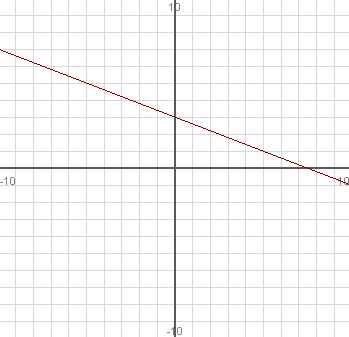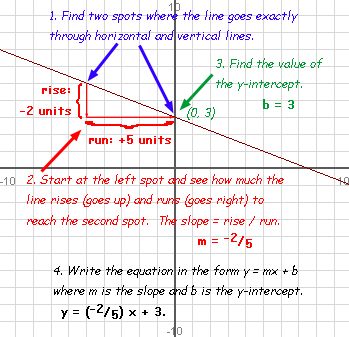SEARCH HOMEMath Central Quandaries & QueriesQuestion from ken, a parent: I have a graph and it is asking me to find the slope of each line, and then write its equation.   the graph show a negative slope.  Would appreciate the answer if you could show me how to do this question. Kind regards:  KenHi Ken. I can show you an example of how to do this.

See the example graph on the right (it is also one with a negative slope):There is a four-step process to determine the equation of the line, with an optional fifth step if you need to put the equation in "standard form".

1. Find two spots on the line where it crosses exactly on the intersection of horizontal and vertical lines of the graph paper. This takes the guesswork out of the problem.
2. Starting with the leftmost point, see how far up (positive) or down (negative) the line goes in reaching the other point. That is the "rise". Also see how many units rightwards you need to go to get there. That is the run. The slope, which we call by the variable "m" equals the rise divided by the run.
3. Find the spot where the line crosses the y-axis. This is called the y-intercept. We use the letter b to represent the y-intercept.
4. Now you have enough information to write the equation in y = mx + b form. Just substitute in the values of m and b.

Often, these questions ask you to put the equation in "standard form" to complete the problem. This is the form Ax + By = C, where A, B, and C are all whole numbers and A is positive.

For this question, we'd multiply both sides by 5, then move the x term to the left side:

y = (-2/5) x + 3
5y = -2 x + 15
2x + 5y = 15.

That would be the final answer.

Cheers,
Stephen La Rocque.Math Central is supported by the University of Regina and The Pacific Institute for the Mathematical Sciences.